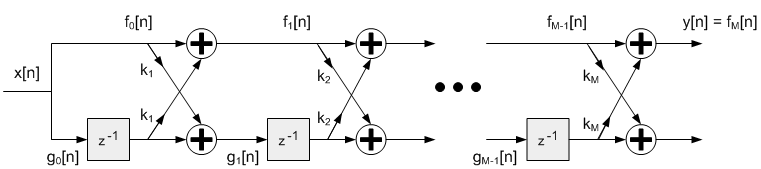Project Ne10 An open, optimized software library for the ARM architecture.
Finite Impulse Response (FIR) Lattice Filters

## Functions

ne10_result_t ne10_fir_lattice_init_float (ne10_fir_lattice_instance_f32_t *S, ne10_uint16_t numStages, ne10_float32_t *pCoeffs, ne10_float32_t *pState)
Initialization function for the floating-point FIR lattice filter. More...

void ne10_fir_lattice_float_c (const ne10_fir_lattice_instance_f32_t *S, ne10_float32_t *pSrc, ne10_float32_t *pDst, ne10_uint32_t blockSize)
Specific implementation of ne10_fir_lattice_float using plain C. More...

void ne10_fir_lattice_float_neon (const ne10_fir_lattice_instance_f32_t *S, ne10_float32_t *pSrc, ne10_float32_t *pDst, ne10_uint32_t blockSize) asm("ne10_fir_lattice_float_neon")
Specific implementation of ne10_fir_lattice_float using NEON SIMD capabilities. More...

## Variables

void(* ne10_fir_lattice_float )(const ne10_fir_lattice_instance_f32_t *S, ne10_float32_t *pSrc, ne10_float32_t *pDst, ne10_uint32_t blockSize)
Processing function for the floating-point FIR lattice filter. More...

## Detailed Description

This set of functions implements Finite Impulse Response (FIR) lattice filters for floating-point data types. Lattice filters are used in a variety of adaptive filter applications. The filter structure is feedforward and the net impulse response is finite length. The functions operate on blocks of input and output data and each call to the function processes `blockSize` samples through the filter. `pSrc` and `pDst` point to input and output arrays containing `blockSize` values.
Algorithm:Finite Impulse Response Lattice filter
The following difference equation is implemented:
```     f0[n] = g0[n] = x[n]
fm[n] = fm-1[n] + km * gm-1[n-1] for m = 1, 2, ...M
gm[n] = km * fm-1[n] + gm-1[n-1] for m = 1, 2, ...M
y[n] = fM[n]
```
`pCoeffs` points to tha array of reflection coefficients of size `numStages`. Reflection Coefficients are stored in the following order.
```     {k1, k2, ..., kM}
```
where M is number of stages
`pState` points to a state array of size `numStages`. The state variables (g values) hold previous inputs and are stored in the following order.
```     {g0[n], g1[n], g2[n] ...gM-1[n]}
```
The state variables are updated after each block of data is processed; the coefficients are untouched.
Instance Structure
The coefficients and state variables for a filter are stored together in an instance data structure. A separate instance structure must be defined for each filter. Coefficient arrays may be shared among several instances while state variable arrays cannot be shared. There are separate instance structure declarations for each of the 3 supported data types.
Initialization Functions
There is also an associated initialization function for each data type. The initialization function performs the following operations:
• Sets the values of the internal structure fields.
• Zeros out the values in the state buffer.
Use of the initialization function is optional. However, if the initialization function is used, then the instance structure cannot be placed into a const data section. To place an instance structure into a const data section, the instance structure must be manually initialized. Set the values in the state buffer to zeros and then manually initialize the instance structure as follows:
```*ne10_iir_lattice_instance_f32_t S = {numStages, pState, pCoeffs};
```
where `numStages` is the number of stages in the filter; `pState` is the address of the state buffer; `pCoeffs` is the address of the coefficient buffer.
Fixed-Point Behavior
Care must be taken when using the fixed-point versions of the FIR Lattice filter functions. In particular, the overflow and saturation behavior of the accumulator used in each function must be considered. Refer to the function specific documentation below for usage guidelines.

## Function Documentation

 void ne10_fir_lattice_float_c ( const ne10_fir_lattice_instance_f32_t * S, ne10_float32_t * pSrc, ne10_float32_t * pDst, ne10_uint32_t blockSize )

Specific implementation of ne10_fir_lattice_float using plain C.

Definition at line 929 of file NE10_fir.c.

 void ne10_fir_lattice_float_neon ( const ne10_fir_lattice_instance_f32_t * S, ne10_float32_t * pSrc, ne10_float32_t * pDst, ne10_uint32_t blockSize )

Specific implementation of ne10_fir_lattice_float using NEON SIMD capabilities.

 ne10_result_t ne10_fir_lattice_init_float ( ne10_fir_lattice_instance_f32_t * S, ne10_uint16_t numStages, ne10_float32_t * pCoeffs, ne10_float32_t * pState )

Initialization function for the floating-point FIR lattice filter.

Parameters
 [in] *S points to an instance of the floating-point FIR lattice structure. [in] numStages number of filter stages. [in] *pCoeffs points to the coefficient buffer. The array is of length numStages. [in] *pState points to the state buffer. The array is of length numStages.

Definition at line 217 of file NE10_fir_init.c.

## Variable Documentation

 void(* ne10_fir_lattice_float) (const ne10_fir_lattice_instance_f32_t *S, ne10_float32_t *pSrc, ne10_float32_t *pDst, ne10_uint32_t blockSize)

Processing function for the floating-point FIR lattice filter.

Parameters
 [in] *S points to an instance of the floating-point FIR lattice structure. [in] *pSrc points to the block of input data. [out] *pDst points to the block of output data [in] blockSize number of samples to process.

Definition at line 180 of file NE10_init_dsp.c.